# CAT Geometry Questions PDF [Most Important with Solutions]

0
227

Geometry is one of the key topics in the CAT Quant section. Over the past few years, CAT Geometry questions have made a recurrent appearance in the Quant section. You can expect around 1-2 questions in the 22-question format of the CAT Quant section. If you’re new to this section, you can check out these CAT Geometry Questions from the CAT previous year papers. In this article, we will look into some very important Geometry questions PDF(with solutions) for CAT. You can also download these CAT Geometry questions with detailed solutions, which also include important tricks to solve these questions.

Question 1: In a triangle ABC, the lengths of the sides AB and AC equal 17.5 cm and 9 cm respectively. Let D be a point on the line segment BC such that AD is perpendicular to BC. If AD = 3 cm, then what is the radius (in cm) of the circle circumscribing the triangle ABC?

a) 17.05

b) 27.85

c) 22.45

d) 32.25

e) 26.25

Solution: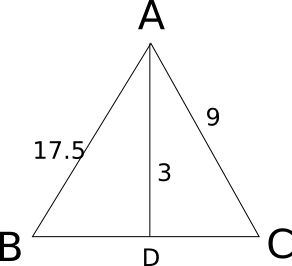Let x be the value of third side of the triangle. Now we know that Area = 17.5*9*x/(4*R), where R is circumradius.

Also Area = 0.5*x*3 .

Equating both, we have 3 = 17.5*9 / (2*R)

=> R = 26.25.

Question 2: Consider obtuse-angled triangles with sides 8 cm, 15 cm and x cm. If x is an integer then how many such triangles exist?

a) 5

b) 21

c) 10

d) 15

e) 14

Solution:

For obtuse-angles triangle, $c^2 > a^2 + b^2$ and c < a+b
If 15 is the greatest side, 8+x > 15 => x > 7 and $225 > 64 + x^2$ => $x^2$ < 161 => x <= 12
So, x = 8, 9, 10, 11, 12
If x is the greatest side, then 8 + 15 > x => x < 23
$x^2 > 225 + 64 = 289$ => x > 17
So, x = 18, 19, 20, 21, 22
So, the number of possibilities is 10

Question 3: Consider a square ABCD with midpoints E, F, G, H of AB, BC, CD and DA respectively. Let L denote the line passing through F and H. Consider points P and Q, on L and inside ABCD such that the angles APD and BQC both equal 120°. What is the ratio of the area of ABQCDP to the remaining area inside ABCD?

a) $\frac{4 \sqrt 2}{3}$

b) $2 + \sqrt{3}$

c) $\frac{10-3\sqrt{3}}{9}$

d) $1+\frac{1}{\sqrt3}$

e)  $2\sqrt{3}-1$

Solution: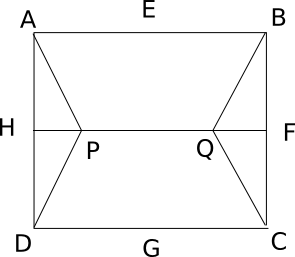Consider side of square as 10 units. So HD=5 and HP=$\frac{5}{\sqrt3}$ . So now area of triangle HPD=$\frac{12.5}{\sqrt3}$. Also Area APD=Area BQC=2*AreaHPD=$\frac{25}{\sqrt3}$. So numerator of required answer is $100-\frac{50}{\sqrt3}$ and denominator as $\frac{50}{\sqrt3}$. Solving we get answer as $2\sqrt{3}-1$.

Question 4: Two circles, both of radii 1 cm, intersect such that the circumference of each one passes through the centre of the other. What is the area (in sq. cm.) of the intersecting region?

a) $\frac{\pi}{3}-\frac{\sqrt 3}{4}$

b) $\frac{2\pi}{3}+\frac{\sqrt 3}{2}$

c) $\frac{4\pi}{3}-\frac{\sqrt 3}{2}$

d) $\frac{4\pi}{3}+\frac{\sqrt 3}{2}$

e) $\frac{2\pi}{3}-\frac{\sqrt 3}{2}$

Solution: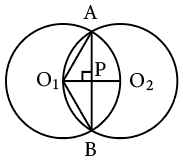The circumferences of the two circle pass through each other’s centers. Hence, O1A = O1B=O1O2 = 1cm

By symmetry, the line joining the two centres would be bisect AB and would be bisected by AB. As the line joining the center to the midpoint of a chord is perpendicular to the chord, O1O2 and AB are perpendicular bisectors of each other. Suppose they intersect at point P.

O1P = Half of O1O2 = 1/2 cm
So, the angle AO1P = 60 degrees as cos 60 = 1/2
By symmetry, BO1P = 60 degrees.
So, angle AO1B= 120 degrees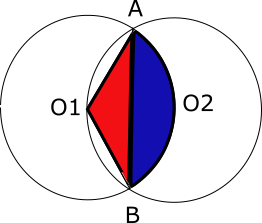In the above, the required area is 2 times A(segment ABO2)(blue region). And A(segment ABO2)(blue region) = A(sector O2AO1B)(blue + red) – A(triangleO1AB )(red)

Area of sector = 120°/360° * $\pi * 1^2$ = $\pi/3$

Area of triangle = 1/2 * b * h = 1/2 * (2* 1 cos 30°) * (1/2) = √3/4

Hence, required area = $\frac{\pi}{3}-\frac{\sqrt 3}{4}$ . Hence so the required area is 2 times the above value which is $\frac{2\pi}{3}-\frac{\sqrt 3}{2}$

Question 5: In the figure below, ABCDEF is a regular hexagon and $\angle{AOF}$ = 90° . FO is parallel to ED. What is the ratio of the area of the triangle AOF to that of the hexagon ABCDEF?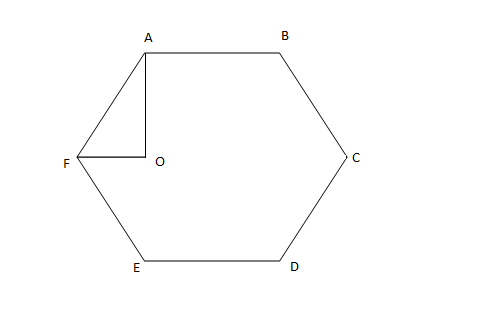a) 1/12

b) 1/6

c) 1/24

d) 1/18

Solution: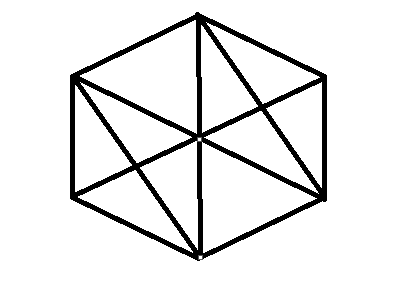When the hexagon is divided into number of similar triangle AOF we get 12 such triangles . Hence required ratio of area is 1/12.
Alternate Approach :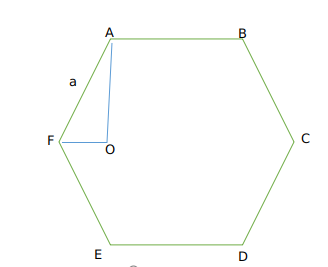Let us take side of the hexagon as a
Now We get AF = a
Now As OF || ED
Angle OFE +Angle E = 180
We know Angle E =120
So angle OFE=60 degrees.
Now therefore Angle AFO=120-60=60 degrees
Now in triangle AFO
Cos60=$\frac{OF}{AF}$
$\frac{1}{2}=\frac{OF}{AF}$
We get OF = $\frac{a}{2}$
Now therefore area of triangle AOF =$\frac{1}{2}\times\ AF\times\ OF\times\ \sin60$
So we get $\frac{1}{2}\times\ a\times\ \frac{a}{2}\times\ \frac{\sqrt{\ 3}}{2}$
=$\frac{\sqrt{\ 3}}{8}a^2$     (1)
Now area of hexagon = $6\times\ \frac{\sqrt{\ 3}}{4}a^2$      (2)
Dividing (1) and (2) we get ratio as $\frac{1}{12}$
Hence option A is the correct answer.

Question 6: In the figure below, AB is the chord of a circle with center O. AB is extended to C such that BC = OB. The straight line CO is produced to meet the circle at D. If $\angle{ACD}$ = y degrees and $\angle{AOD}$ = x degrees such that x = ky, then the value of k is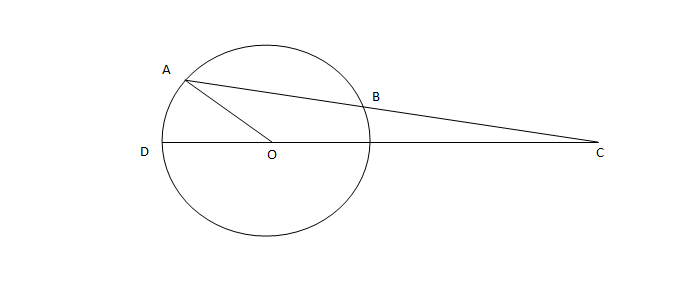a) 3

b) 2

c) 1

d) None of the above.

Solution: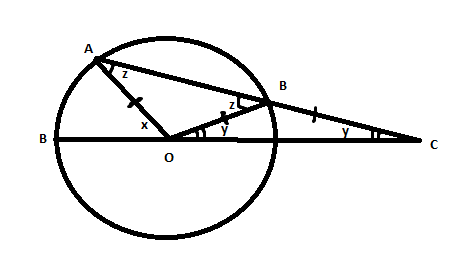Since Angle BOC = Angle BCO = y.

Angle OBC = 180-2y .

Hence Angle ABO =  z = 2y = Angle OAB.

Now since x is exterior angle of triangle AOC .

We have x = z + y = 3y.

Hence option A.

Question 7: A father and his son are waiting at a bus stop in the evening. There is a lamp post behind them. The lamp post, the father and his son stand on the same straight line. The father observes that the shadows of his head and his son’s head are incident at the same point on the ground. If the heights of the lamp post, the father and his son are 6 metres, 1.8 metres and 0.9 metres respectively, and the father is standing 2.1 metres away from the post then how far (in metres) is son standing form his father?

a) 0.9

b) 0.75

c) 0.6

d) 0.45

Solution:

The three triangles are similar.

Let the distance of the tip of the shadow from the child be y. Let the child be standing at distance x from the father.

So, 6/(2.1+x+y) = 1.8/(x+y) = 0.9/y

=> 2y = x+y => x = y

=> 6/(2.1 + 2x) = 0.9/x

=> 6x = 0.9*(2.1+2x)

=> 6x = 1.89 + 1.8x

=> 4.2x = 1.89

=> x = 1.89/4.2 = 0.45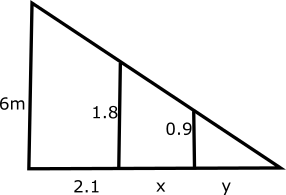Question 8: If the lengths of diagonals DF, AG and CE of the cube shown in the adjoining figure are equal to the three sides of a triangle, then the radius of the circle circumscribing that triangle will be?a) equal to the side of the cube

b) $\sqrt 3$ times the side of the cube

c) 1/$\sqrt 3$ times the side of the cube

d) impossible to find from the given information

Solution:

Consider side of the cube as x.

So diagonal will be of length $\sqrt{3}$ * x.

Now if diagonals are side of equilateral triangle we get area = 3*$\sqrt{3}*x^2$ /4 .

Also in a triangle

4 * Area * R = Product of sides

4* 3*$\sqrt{3}*x^2$ /4 * R = .3*$\sqrt{3}*x^3$

R = x

Question 9: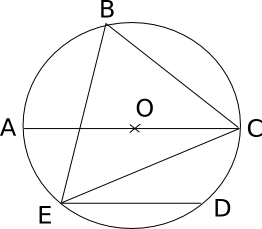In the adjoining figure, chord ED is parallel to the diameter AC of the circle. If angle CBE = 65°, then what is the value of angle DEC?

a) 35

b) 55

c) 45

d) 25

Solution: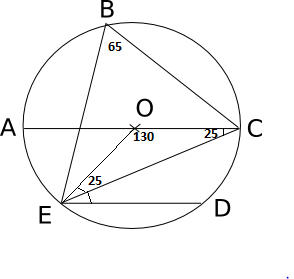If EBC = 65 then EOC = 130 then OEC = OCE = 25. NOw since OC and ED are parallel we have OCE = OED = 25. Hence option D.

Question 10: What is the distance in cm between two parallel chords of lengths 32 cm and 24 cm in a circle of radius 20 cm?

a) 1 or 7

b) 2 or 14

c) 3 or 21

d) 4 or 28

Solution: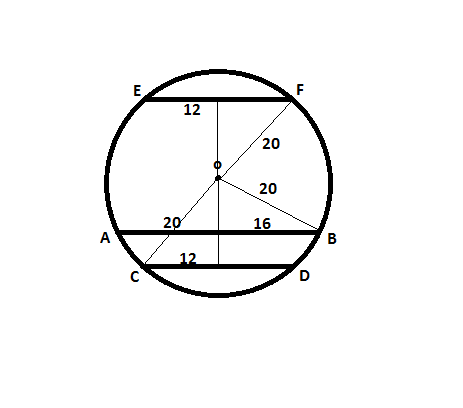The distances of the chords from the center are 12 cm and 16 cm respectively.

If the chords lie on the same side of the center, the distance between the chords is 4 cm, if they lie on opposite sides of the center, the distance between them is 28 cm.

Question 11: Two identical circles intersect so that their centres, and the points at which they intersect, form a square of side 1 cm. The area in sq. cm of the portion that is common to the two circles is

a) $\pi$/4

b) $\pi$/2-1

c) $\pi$/5

d) $\sqrt\pi-1$

Solution: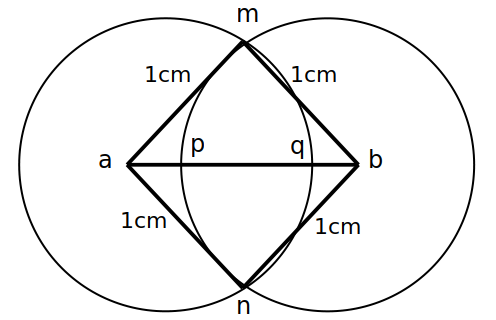We know that quad ambn is a square of side 1.

Area of the sector a-mqn is $\frac{90}{360}* \pi *1*1$ = $\frac{\pi }{4}$.

Area of square = 1*1 = 1

Area of common portion = 2 * Area of sector – Area of square

= 2 * $\frac{\pi }{4}$ – 1 =  $\frac{\pi }{2}$ – 1

Question 12: In the following figure, the diameter of the circle is 3 cm. AB and MN are two diameters such that MN is perpendicular to AB. In addition, CG is perpendicular to AB such that AE:EB = 1:2, and DF is perpendicular to MN such that NL:LM = 1:2. The length of DH in cm is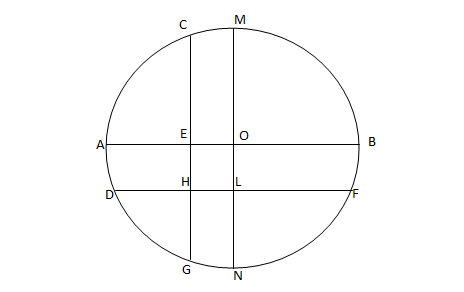a) $2\sqrt2 – 1$

b) $(2\sqrt2 – 1)/2$

c) $(3\sqrt2 – 1)/2$

d) $(2\sqrt2 – 1)/3$

Solution:

Let EO = x, So, AE = 1.5 – x
AE : EB = 1:2 => x = 1/2

(1.5-x):(1.5+x) = 1:2.

x=0.5.

So, EO = 0.5
Similarly, OL = 0.5
Now, EOLH is a parallelogram and EO = OL = 0.5
In triangle DOL, DO = radius = 1.5 and OL = 0.5
So, DL = $\sqrt2$
=> DH = $(2\sqrt2 – 1)/2$

Question 13: A square, whose side is 2 m, has its corners cut away so as to form an octagon with all sides equal. Then the length of each side of the octagon, in metres, is

a) $\frac{\sqrt 2}{\sqrt 2 +1}$

b) $\frac{2}{\sqrt 2 + 1}$

c) $\frac{2}{\sqrt 2 – 1}$

d) $\frac{\sqrt 2}{\sqrt 2-1}$

Solution:

Let the length of each side of the octagon be x.

So, length of the square will be x+2*(x/√2) = 2

=> x(1+√2) = 2 => x = 2/(1+√2)

Question 14: In the given diagram, ABCD is a rectangle with AE = EF = FB. What is the ratio of the areas of CEF and that of the rectangle?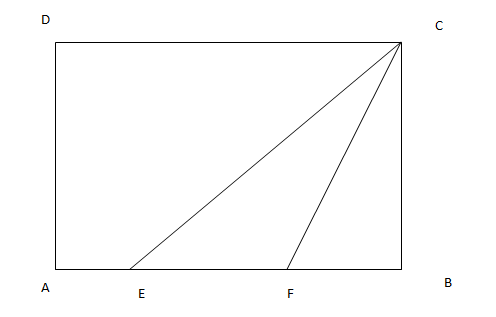a) $\frac{1}{6}$

b) $\frac{1}{8}$

c) $\frac{1}{9}$

d) None of these

Solution:

Let the length of AB be 3X and the length of AD (and BC) be Y.

As the length of AB = 3X, AE = EF = FB =X

So, the area of the rectangle ABCD is length * breadth = 3X * Y = 3XY

The area of triangle CEF = 1/2 * base * height = 1/2 * EF * BC = 1/2*X*Y

So, required ratio = 1/2 : 3 = 1:6

Question 15: A ladder leans against a vertical wall. The top of the ladder is 8 m above the ground. When the bottom of the ladder is moved 2 m farther away from the wall, the top of the ladder rests against the foot of the wall. What is the length of the ladder?

a) 10 m

b) 15 m

c) 20 m

d) 17 m

Solution:

When the ladder is moved 2 m away from the wall, the top of the ladder rests against the foot of the wall. So, the hypotenuse is 2 m more than the second side of the right triangle. The other non-hypotenuse side is 8 m. So, $8^2 + x^2 = (x+2)^2$

=> x = 15 m and length of the ladder = x+2 = 17 m

Question 16: Two sides of a plot measure 32 m and 24 m and the angle between them is a perfect right angle. The other two sides measure 25 m each and the other three angles are not right angles. What is the area of the plot?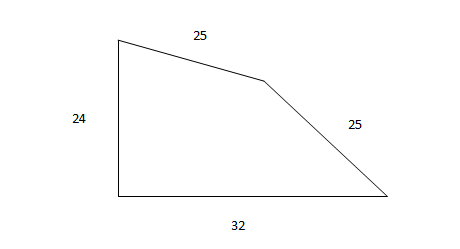a) 768 $m^2$

b) 534 $m^2$

c) 696.5 $m^2$

d) 684 $m^2$

Solution:

Length of the diagonal of the right triangle is 40.

The height of the isosceles triangle formed, with 40 as its base is 15.
So, area = (1/2 * 32 * 24) + (1/2 * 40 * 15) = 384 + 300 = 684 $m^2$

Question 17: In triangle DEF shown below, points A, B and C are taken on DE, DF and EF respectively such that EC = AC and CF = BC. If angle D equals 40 degress , then angle ACB is ?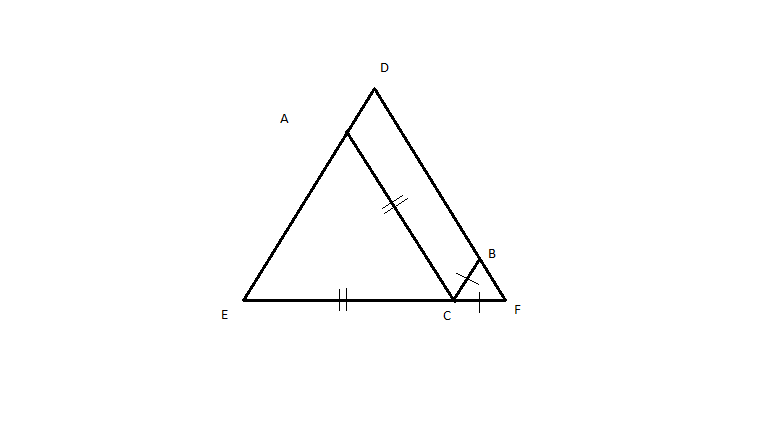a) 140

b) 70

c) 100

d) None of these

Solution:

Let angle EAC = x, so angle AEC = x and angle ACE = 180-2x
Let angle FBC = y, so angle BFC = y and angle BCF = 180-2y
So, angle ACB = 180-(180-2x+180-2y) = 2(x+y) – 180
x+y = 180 – 40 = 140
So, angle ACB = 280 – 180 = 100

Question 18: Consider a circle with unit radius. There are 7 adjacent sectors, S1, S2, S3,….., S7 in the circle such that their total area is (1/8)th of the area of the circle. Further, the area of the $j^{th}$ sector is twice that of the $(j-1)^{th}$ sector, for j=2, …… 7. What is the angle, in radians, subtended by the arc of S1 at the centre of the circle?

a) $\pi/508$

b) $\pi/2040$

c) $\pi/1016$

d) $\pi/1524$

Solution:

Now area of 1st sector = $\pi * r^2 * \frac{x}{360}$ where x – angle subtended at center

Now the next sector will have 2x as the angle, and similarly angles will be in GP with ratio = 2.

Sum of areas of all 7 sectors = $\frac{127*x* \pi * r^2}{360}$ which is equal to $\frac{\pi * r^2}{8}$

We get x = $\frac{360}{8*127}$

Now if converted in radians we get  x = $\pi/508$.

Question 19: If a,b,c are the sides of a triangle, and $a^2 + b^2 +c^2 = bc + ca + ab$, then the triangle is:

a) equilateral

b) isosceles

c) right angled

d) obtuse angled

Solution:

$(a+b+c)^2 = a^2 + b^2 + c^2 + 2(ab + bc + ca) => 3(a^2 + b^2 + c^2)$

This is possible only if a = b = c.

So, the triangle is an equilateral triangle.

Question 20: In the figure given below, ABCD is a rectangle. The area of the isosceles right triangle ABE = 7 $cm^2$ ; EC = 3(BE). The area of ABCD (in $cm^2$) is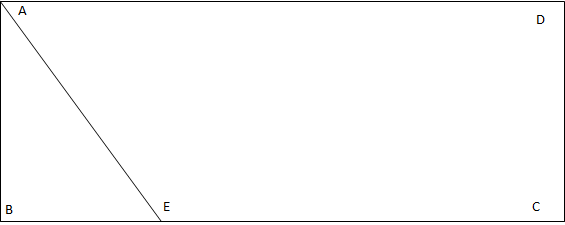a) 21 $cm^2$

b) 28 $cm^2$

c) 42 $cm^2$

d) 56 $cm^2$

Solution:Let AB = BE = x

Area of triangle ABE = $x^2/2$ = 14; we get x = $\sqrt{14}$

So we have side BC = 4*$\sqrt{14}$

Now area is AB*BC = 14 *4 = 56 $cm^2$

Question 21: The area of the triangle whose vertices are (a,a), (a + 1, a + 1) and (a + 2, a) is
[CAT 2002]

a) $a^3$

b) $1$

c) $2a$

d) $2^{1/2}$

Solution:

The triangle we have is :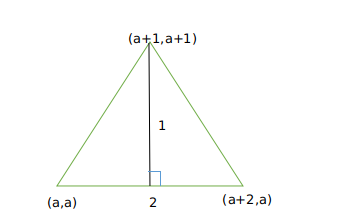The length of three sides is $\sqrt 2, \sqrt 2$ and $2$.
This is a right-angled triangle.
Hence, it’s area equals $1/2 * \sqrt 2 * \sqrt 2 = 1$
So, the correct answer is b)
Alternate Approach :
Area of triangle = $\frac{1}{2}\times\ base\times\ height$
So we get $\frac{1}{2}\times2\times\ 1$=1 square units

Question 22: Instead of walking along two adjacent sides of a rectangular field, a boy took a short cut along the diagonal and saved a distance equal to half the longer side. Then the ratio of the shorter side to the longer side is

a) 1/2

b) 2/3

c) 1/4

d) 3/4

Solution: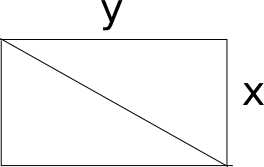Let x be the shorter side and y be the longer one. The shortcut route would be of length $\sqrt{x^2+y^2}$. According to given condition we know that (x+y)- $\sqrt{x^2+y^2}$ = y/2 . Solving, we get 1=(x/y)+(1/4)

=> x : y = 3 : 4. Hence option D is the correct answer.

Question 23: In the following figure, ACB is a right-angled triangle. AD is the altitude. Circles are inscribed within the triangle ACD and triangle BCD. P and Q are the centers of the circles. The distance PQ is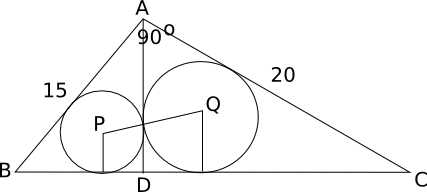The length of AB is 15 m and AC is 20 m

a) 7 m

b) 4.5 m

c) 10.5 m

d) 6 m

Solution: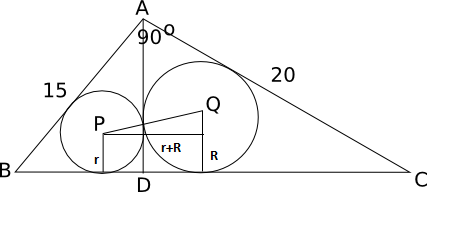By Pythagoras theorem we get BC = 25 . Let BD = x;Triangle ABD is similar to triangle CBA => AD/15 = x/20 and also triangle ADC is similar to triangle ACB=> AD/20 = (25-x)/15. From the 2 equations, we get x = 9 and DC = 16

We know that AREA = (semi perimeter ) * inradius

For triangle ABD, Area = 1/2 x BD X AD = 1/2 x 12 x 9 = 54 and semi perimeter = (15 + 9 + 12)/2 = 18. On using the above equation we get, inradius, r = 3.

PQ = R + r = 7 cm

Question 24: Let the consecutive vertices of a square S be A,B,C &D. Let E,F & G be the mid-points of the sides AB, BC & AD respectively of the square. Then the ratio of the area of the quadrilateral EFDG to that of the square S is nearest to

a) 1/2

b) 1/3

c) 1/4

d) 1/8

Solution: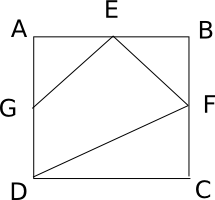Let the side of the square be 1 cm

So, area of triangle AGE = 1/2 * 1/2 * 1/2 = 1/8

Similarly, area of triangle EBF = 1/8

Area of triangle DFC = 1/2 * 1 * 1/2 = 1/4

Area of the square = 1*1 = 1

So, area of the quadrilateral = 1 – (1/8 + 1/8 + 1/4) = 1 – 1/2 = 1/2

Option a) is the correct answer.

Question 25: A circle is inscribed in a given square and another circle is circumscribed about the square. What is the ratio of the area of the inscribed circle to that of the circumscribed circle?

a) 2 : 3

b) 3 : 4

c) 1 : 4

d) 1 : 2

Solution:

As we know that area of the circle is directly proportional to the square of its radius.
Hence $\frac{A_{ic}}{A_{cc}} = \frac{\frac{x^2}{4}}{\frac{x^2}{2}}$
Where $x$ is side of square (say), ic is inscribed circle with radius $\frac{x}{2}$, cc is circumscribed circle with radius $\frac{x}{\sqrt{2}}$
So ratio will be 1:2

Question 26: A one rupee coin is placed on a table. The maximum number of similar one rupee coins which can be placed on the table, around it, with each one of them touching it and only two others is

a) 8

b) 6

c) 10

d) 4

Solution:

Considering the centre coin which is denoted by  C1.

The surrounding coins are of equal radius as the centre coin. Since each coin is placed next to each other and considering the total number of coins = n.

The pairs formed by the coins which touch the consecutive coins is given by :

(1, 2) , (2, 3) ……….. (n, 1)

The triangles formed by joining the centres of all the consecutive circles at the centre of C1 = 360 degrees.

If we join centres of 2 outer circles with the centre circle, it will make an equilateral triangle.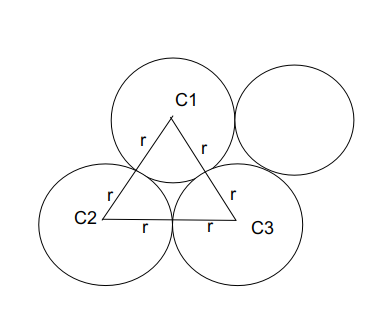A single pair forms 60 degrees as it forms an equilateral triangle. Hence $\frac{360}{n}=\ 60$

n = 6

Question 27: If ABCD is a square and BCE is an equilateral triangle, what is the measure of ∠DEC?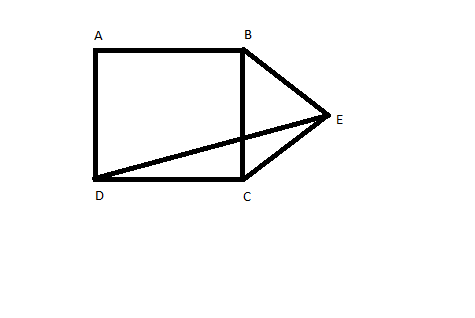a) 15°

b) 30°

c) 20°

d) 45°

Solution:

According to given diagram, as triangle BCE is equilateral CE=BE=DC
Hence angle CDE = angle CED

So angle DEC = $\frac{180-150}{2} = 15$
Question 28: In triangleABC, ∠B is a right angle, AC = 6 cm, and D is the mid-point of AC. The length of BD is

a) 4cm

b) 6 cm

c) 3cm

d) 3.5cm

Solution:

A circle is circumscribed to triangle ABC.

For this circle D will be centre of circle, and AD , DC , BD will radius of this circle.

Question 29: The figure shows the rectangle ABCD with a semicircle and a circle inscribed inside in it as shown. What is the ratio of the area of the circle to that of the semicircle?

a) $(\sqrt2 -1)^{2}:1$

b) $2(\sqrt{2} -1)^2 :1$

c) $(\sqrt2-1)^2 :2$

d) None of these

Solution:

Let the center be O and the point at which the semicircle intersects CD be P.

Let the radius of the semicircle be R and the circle be r.

OP = R and OC = R$\sqrt{2}$

OC – OT = CC’ – TC’

$R\sqrt{2} – R – 2r$ = $r\sqrt{2} – r$

=> $R\sqrt{2} – R$ = $r\sqrt{2} + r$

=> r = $\frac{(\sqrt{2}-1)R}{\sqrt{2}+1}$

=> r = $(\sqrt{2}-1)^2$R

Ratio of areas will be $r^2 : \frac{R^2}{2}$ = $2(\sqrt{2}-1)^4$ : 1

Question 30: The sum of the areas of two circles, which touch each other externally, is $153\pi$. If the sum of their radii is 15, find the ratio of the larger to the smaller radius.

a) 4

b) 2

c) 3

d) None of these

Solution:

Given:
$\pi((r_1)^2 + (r_2)^2) = 153\pi$
So
$(r_1)^2 + (r_2)^2 = 153$
Or $((r_1) + (r_2))^2 – 2(r_1)(r_2) = 153$
Or  $(r_1)(r_2) = 36$ and $(r_1) + (r_2) = 15$
$r_1 = 12$
$r_2 = 3$
Ratio = 4

Videos for Important Geometry Questions | CAT Online Coaching